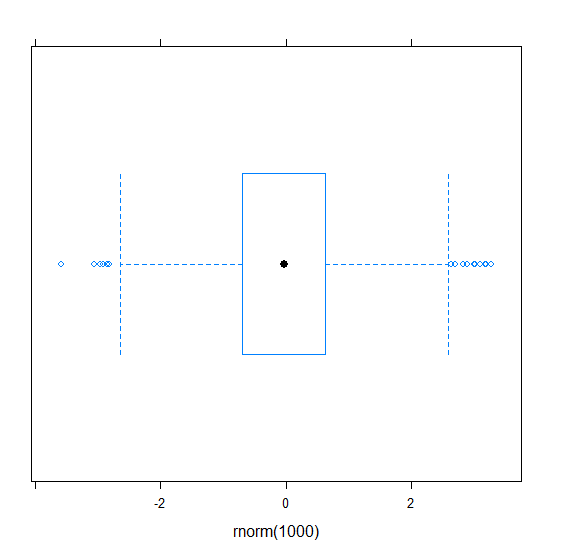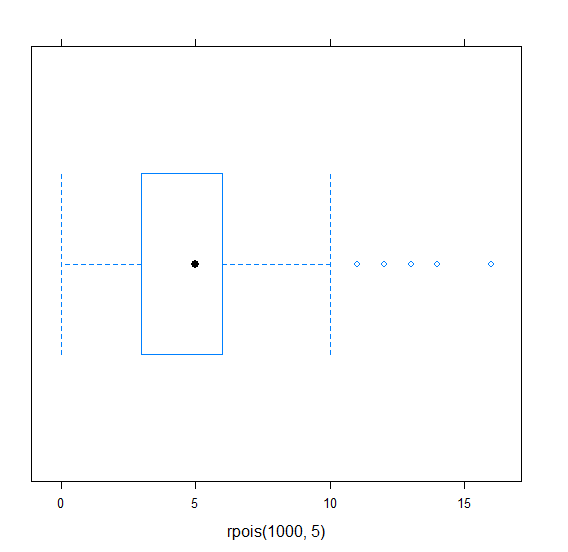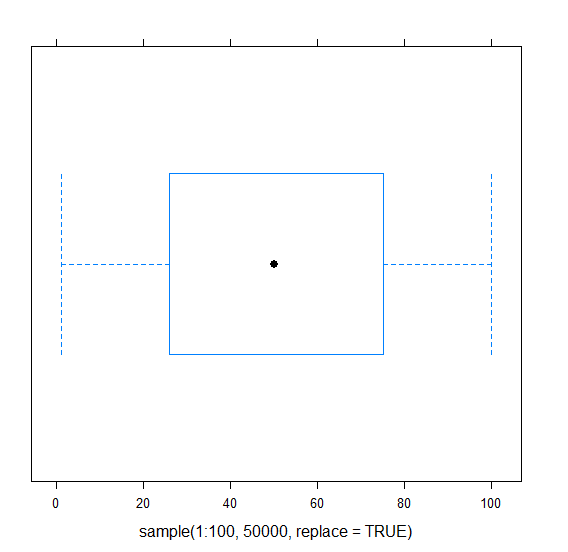# How to create transparent boxplot in R?

Be default, the boxplot created in base R or by using ggplot2 are not transparent in nature. If we want to create a transparent boxplot then we can use bwplot function from lattice package.

For Example, if we have a vector called X then we can create transparent boxplot of X by using the below command −

bwplot(x)

## Example 1

To create transparent boxplot use the snippet given below −

library(lattice)
bwplot(rnorm(1000))

## Output

If you execute the above given snippet, it generates the following Output −## Example 2

To create transparent boxplot add the following code to the above snippet −

library(lattice)
bwplot(rpois(1000,5))

## Output

If you execute all the above given snippets as a single program, it generates the following Output −## Example 3

To create transparent boxplot add the following code to the above snippet −

library(lattice)
bwplot(sample(1:100,50000,replace=TRUE))


## Output

If you execute all the above given snippets as a single program, it generates the following Output −